# Dynamic Traffic Management System

Description : traffic signals dynamically displays output based upon intensity of traffic across lanes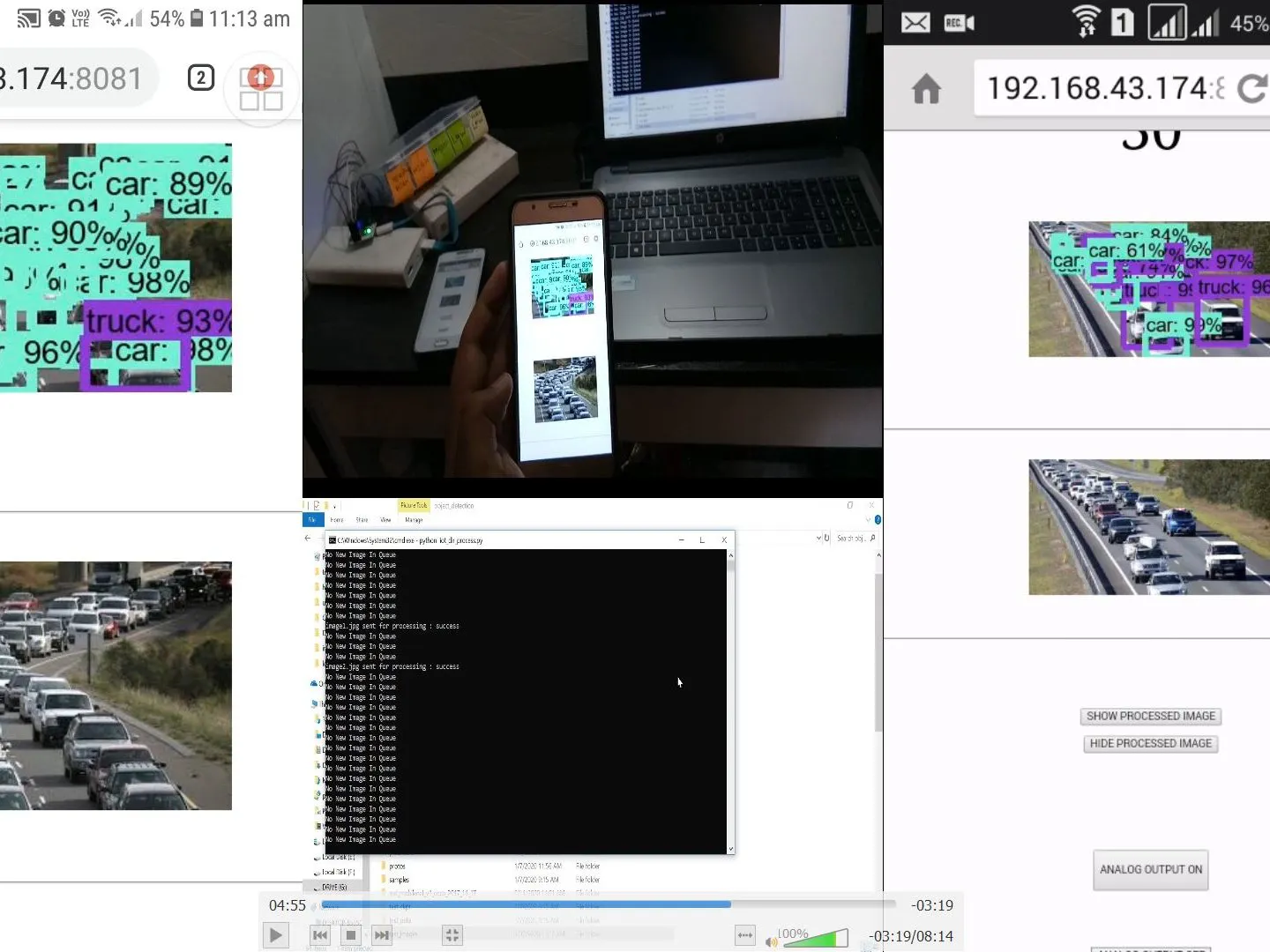## Things used in this project

### Hardware components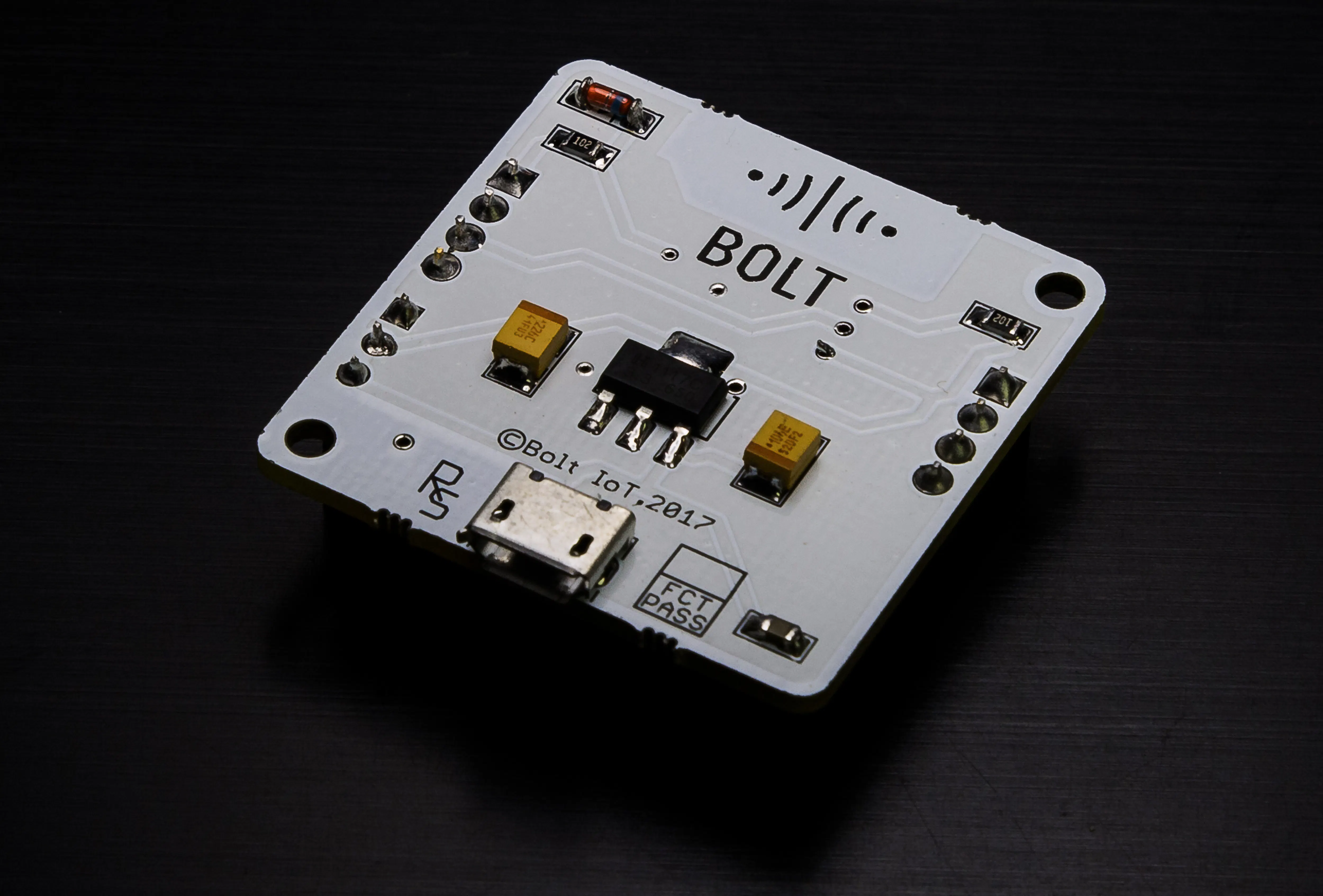Bolt IoT Bolt WiFi Module
×1

### Hand tools and fabrication machines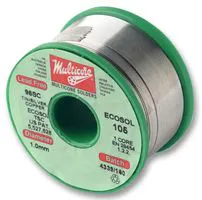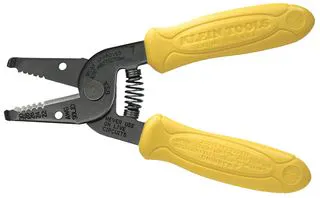Wire Stripper & Cutter, 18-10 AWG / 0.75-4mm² Capacity Wires

## Schematics

### Process Flow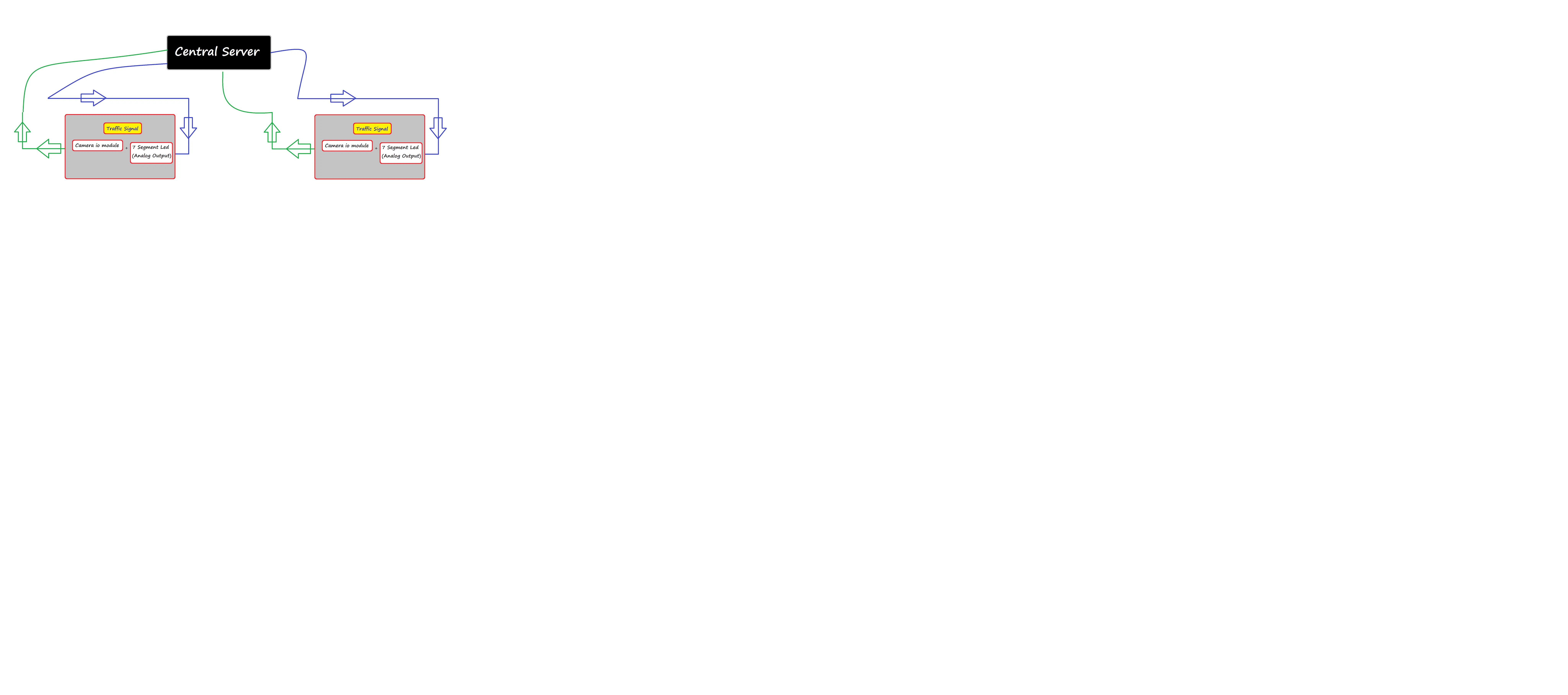## Code

Python
to understand the directory structure of projecct
i am uploading the main ob_detection file which consist of the code go through the directory names and make them and comment uncomment the code as per your need
install ananconda command prompt and python on your system
watch edurekas video on object detection

If this project can work on my system then it will for sure run on your system too but for that go through the code thoroughly
```# coding: utf-8

# # Object Detection Demo
# Welcome to the object detection inference walkthrough!  This notebook will walk you step by step through the process of using a pre-trained model to detect objects in an image. Make sure to follow the [installation instructions](https://github.com/tensorflow/models/blob/master/research/object_detection/g3doc/installation.md) before you start.

# # Imports
import subprocess
import shutil
import numpy as np
import os
import openpyxl
import six.moves.urllib as urllib
import sys
import tarfile
import tensorflow as tf
from pathlib import Path
from matplotlib import pyplot as plt

from PIL import Image

# This is needed since the notebook is stored in the object_detection folder.
sys.path.append("..")
from object_detection.utils import ops as utils_ops

if tf.__version__ < '1.4.0':

#import cv2
#cap = cv2.VideoCapture(0)

# ## Env setup

from utils import label_map_util

from utils import visualization_utils as vis_util

# # Model preparation

# ## Variables
#
# Any model exported using the `export_inference_graph.py` tool can be loaded here simply by changing `PATH_TO_FROZEN_GRAPH` to point to a new .pb file.
#
# By default we use an "SSD with Mobilenet" model here. See the [detection model zoo](https://github.com/tensorflow/models/blob/master/research/object_detection/g3doc/detection_model_zoo.md) for a list of other models that can be run out-of-the-box with varying speeds and accuracies.

single_image_name=sys.argv

# MODEL_NAME = 'faster_rcnn_nas_coco_2018_01_28'
MODEL_NAME = 'ssd_mobilenet_v1_coco_2017_11_17'
MODEL_FILE = MODEL_NAME + '.tar.gz'

# Path to frozen detection graph. This is the actual model that is used for the object detection.
PATH_TO_FROZEN_GRAPH = MODEL_NAME + '/frozen_inference_graph.pb'

# List of the strings that is used to add correct label for each box.
PATH_TO_LABELS = os.path.join('data', 'mscoco_label_map.pbtxt')

NUM_CLASSES = 90

# Check the frozen_inference_graph is exists at path.
# If not ssd_mobilenet_v1_coco_2017_11_17 model is downloaded and frozen_inference_graph.pb moved to path.
if os.path.exists(PATH_TO_FROZEN_GRAPH):

else:
opener = urllib.request.URLopener()
tar_file = tarfile.open(MODEL_FILE)
for file in tar_file.getmembers():
file_name = os.path.basename(file.name)
if 'frozen_inference_graph.pb' in file_name:
tar_file.extract(file, os.getcwd())

# ## Load a (frozen) Tensorflow model into memory.

detection_graph = tf.compat.v1.Graph()
with detection_graph.as_default():
od_graph_def = tf.compat.v1.GraphDef()
with tf.io.gfile.GFile(PATH_TO_FROZEN_GRAPH, 'rb') as fid:
od_graph_def.ParseFromString(serialized_graph)
tf.import_graph_def(od_graph_def, name='')

# Label maps map indices to category names, so that when our convolution network predicts `5`, we know that this corresponds to `airplane`.  Here we use internal utility functions, but anything that returns a dictionary mapping integers to appropriate string labels would be fine

categories = label_map_util.convert_label_map_to_categories(label_map, max_num_classes=NUM_CLASSES, use_display_name=True)
category_index = label_map_util.create_category_index(categories)

# # Detection
(im_width, im_height) = image.size
return np.array(image.getdata()).reshape(
(im_height, im_width, 3)).astype(np.uint8)

def run_inference_for_single_image(image, graph):
with graph.as_default():
with tf.compat.v1.Session() as sess:
# Get handles to input and output tensors
ops = tf.compat.v1.get_default_graph().get_operations()
all_tensor_names = {output.name for op in ops for output in op.outputs}
tensor_dict = {}
for key in [
'num_detections', 'detection_boxes', 'detection_scores',
]:
tensor_name = key + ':0'
if tensor_name in all_tensor_names:
tensor_dict[key] = tf.compat.v1.get_default_graph().get_tensor_by_name(
tensor_name)
# The following processing is only for single image
detection_boxes = tf.squeeze(tensor_dict['detection_boxes'], )
# Reframe is required to translate mask from box coordinates to image coordinates and fit the image size.
real_num_detection = tf.cast(tensor_dict['num_detections'], tf.int32)
detection_boxes = tf.slice(detection_boxes, [0, 0], [real_num_detection, -1])
image_tensor = tf.compat.v1.get_default_graph().get_tensor_by_name('image_tensor:0')

# Run inference
output_dict = sess.run(tensor_dict,
feed_dict={image_tensor: np.expand_dims(image, 0)})

# all outputs are float32 numpy arrays, so convert types as appropriate
output_dict['num_detections'] = int(output_dict['num_detections'])
output_dict['detection_classes'] = output_dict[
'detection_classes'].astype(np.uint8)
output_dict['detection_boxes'] = output_dict['detection_boxes']
output_dict['detection_scores'] = output_dict['detection_scores']
return output_dict

PATH_TO_TEST_IMAGES_DIR = 'test_images'

#TEST_IMAGE_PATHS = [ os.path.join(PATH_TO_TEST_IMAGES_DIR, 'image{}.jpg'.format(i)) for i in range(1,8) ]
# entries = Path('test_images/')
# lis = []
# for ent in entries.iterdir():
#   lis.append(os.path.join(PATH_TO_TEST_IMAGES_DIR,ent.name))

TEST_IMAGE_PATHS = [os.path.join(PATH_TO_TEST_IMAGES_DIR,single_image_name)]

for image_path in TEST_IMAGE_PATHS:
image = Image.open(image_path)
# the array based representation of the image will be used later in order to prepare the
# result image with boxes and labels on it.
#while True:
# Actual detection.
output_dict = run_inference_for_single_image(image_np, detection_graph)
# Visualization of the results of a detection.
# print(category_index)
#print("----------",image_path,"------------")
# print(output_dict)
count = 0
result = {}

for data in output_dict['detection_scores']:
count+=1
if data > 0.5:
#print(data," ",count," ",output_dict['detection_classes'][count-1])
ans = (category_index[output_dict['detection_classes'][count-1]]['name']).upper()
if ans in result.keys():
result[ans]+=1
else:
result[ans]=1

vis_util.visualize_boxes_and_labels_on_image_array(
image_np,
output_dict['detection_boxes'],
output_dict['detection_classes'],
output_dict['detection_scores'],
category_index,
use_normalized_coordinates=True,
line_thickness=8)

def cellval(sheet_obj,i,j,val):
sheet_obj.cell(row=i,column=j).value=val

def updateColumn(clm_lst,sheet_obj):
mclm=sheet_obj.max_column
print(mclm)
for i in range(1,mclm+1):
clm_name=sheet_obj.cell(row=1,column=i).value
if clm_name in clm_lst:
clm_lst.remove(clm_name)

for i in range(1,len(clm_lst)+1):
sheet_obj.cell(row=1,column=mclm+i).value=clm_lst[i-1].upper()

def exl_write(path,result,time,intensity):
sheet_obj = wb_obj.active
sheet_obj.cell(row=1,column=1).value="NAME"
sheet_obj.cell(row=1,column=2).value="TIME"
sheet_obj.cell(row=1,column=3).value="INTENSITY"
clm_lst=[]
for key in result.keys():
clm_lst.append(key)

updateColumn(clm_lst,sheet_obj)
# print(clm_lst)
row_max=sheet_obj.max_row
clm_with_inx={}
# print(sheet_obj.max_column)
for i in range(3,sheet_obj.max_column+1):
clm_with_inx[sheet_obj.cell(row=1,column=i).value]=i

sheet_obj.cell(row=1+row_max,column=2).value=time
sheet_obj.cell(row=1+row_max,column=3).value=intensity
# for k,v in clm_with_inx.items():
#   print(k+" "+str(v))
inx=1+row_max
cellval(sheet_obj,inx,1,image_path[-10:])
for key,val in result.items():
cellval(sheet_obj,inx,clm_with_inx[key],val)

wb_obj.save(path)

def cal_time(result):
car=0
truck=0
bike=0
if "CAR" in result.keys():
car=result["CAR"]
if "TRUCK" in result.keys():
truck=result["TRUCK"]
if "BUS" in result.keys():
truck=truck+result["BUS"]
if "MOTORBIKE" in result.keys():
bike=result["MOTORBIKE"]

time=((81/14.0)*car+(69/14.0)*truck+(30/7.0)*bike)
return round(time)

title=""
for key,val in result.items():
title+=(key+"-"+str(val)+"\n")

##plt.figure(figsize=(16, 9))
#   plt.imshow(image_np)

img = Image.fromarray(image_np)
img.save('output\\output_'+image_path[-10:])
img.save('C:\\xampp\\htdocs\\output\\output_'+image_path[-10:])

time=cal_time(result)
intensity=""
if time<15:
time=15
intensity='VERY LOW TRAFFIC (0-15) seconds\n'
elif time<=60:
intensity='LOW TRAFFIC (15-60) seconds\n'
elif time<=120:
intensity='MEDIUM TRAFFIC (60-120) seconds\n'
elif time<=180:
intensity='HIGH TRAFFIC (120-180) seconds\n'
elif time<=240:
intensity='VERY HIGH TRAFFIC (180-240) seconds\n'
elif time>240:
time=240
intensity='IMMENSE TRAFFIC (>240) seconds\n'

log='logs\\log_'+ image_path[-10:-3]+'txt';
file=open(log,"w")
file.write(str(time)+"\n"+intensity+"\n"+title)
file.close()
exl_write("G:/Tut/models/research/object_detection/history/data.xlsx",result,time,intensity)
# subprocess.Popen('G:/Tut/models/research/object_detection/clean_dir.bat')
# if single_image_name == "image1.jpg":
#   shutil.copy("cp/log_image1.txt","Logs")
#   shutil.copy("cp/output_image1.jpg","output")
#   shutil.copy("cp/output_image1.jpg","C:/xampp/htdocs/output")

# if single_image_name == "image2.jpg":
#   shutil.copy("cp/log_image2.txt","Logs")
#   shutil.copy("cp/output_image2.jpg","output")
#   shutil.copy("cp/output_image2.jpg","C:/xampp/htdocs/output")

shutil.copy("cp/data.xlsx","history")
for ent in TEST_IMAGE_PATHS:
os.remove(ent)

# file=open('history\\data.txt',"a")
# hist="-------------------"
# hist+="\nName : " + image_path[-10:]
# title=title.replace("-","")
# hist+=title
# file.write(hist)
# file.close()
#  plt.title(title, fontdict = {'fontsize' : 50})

'''  cv2.imshow('Image Detction', cv2.resize(image_np, (100,200)))
if cv2.waitKey(25) & 0xFF == ord('q'):
cv2.destroyAllWindows()
break
'''

```

## Credits

### amit bhasita

1 project • 0 followers